# The acid ionization constant for Al(H20)+(aq) is 7.9x106. Calculate the pH of a 0.0523 M solution...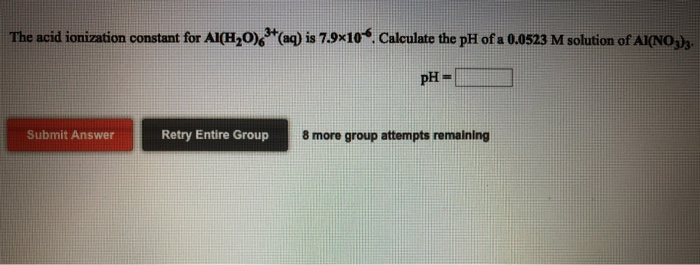The acid ionization constant for Al(H20)+(aq) is 7.9x106. Calculate the pH of a 0.0523 M solution of AINO) pH = Submit Answer Retry Entire Group 8 more group attempts remaining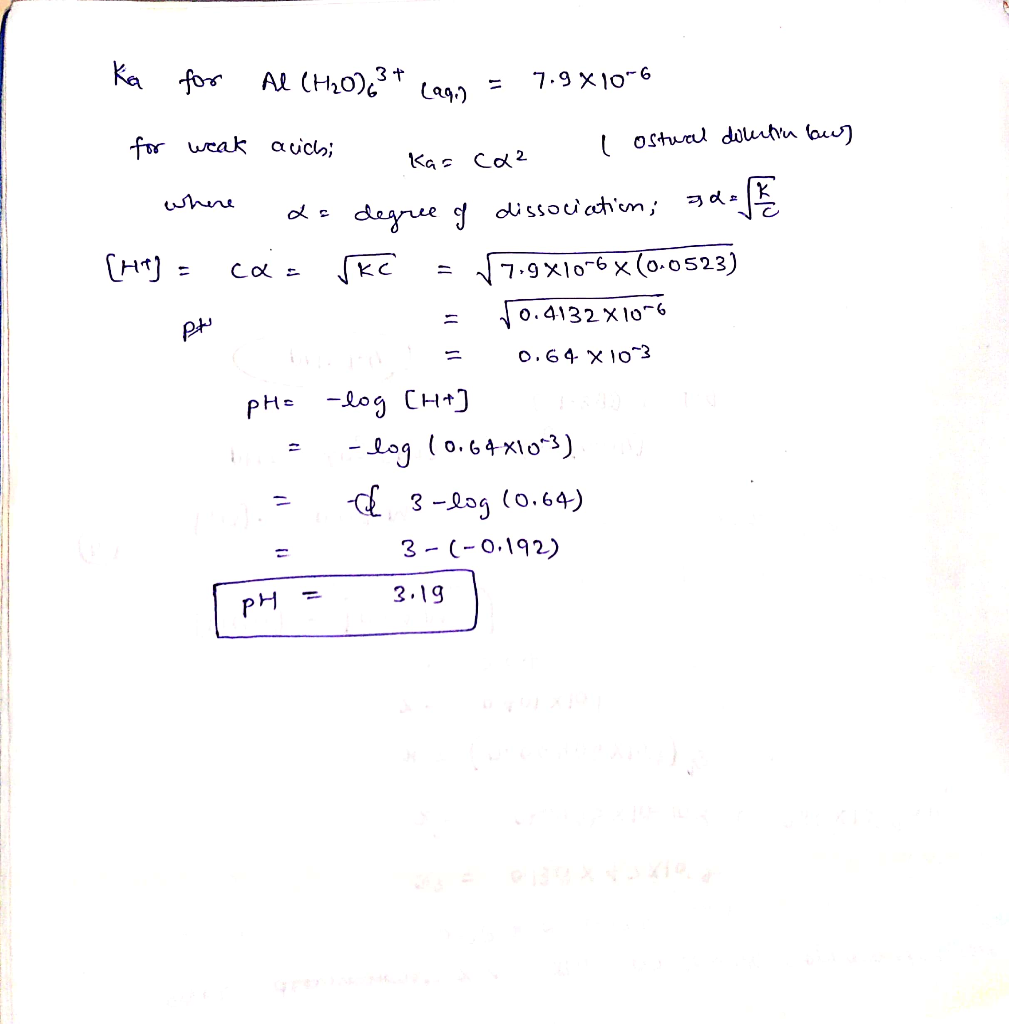#### Earn Coin

Coins can be redeemed for fabulous gifts.

Similar Homework Help Questions
• ### The acid ionization constant for Ga(H20) (a) is 2.5x103 Calculate the pH of a 0.1620 M solution o...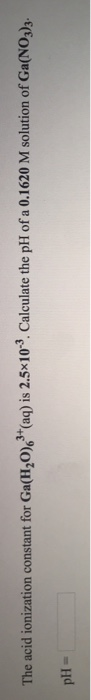The acid ionization constant for Ga(H20) (a) is 2.5x103 Calculate the pH of a 0.1620 M solution of Ga(NO,J)s The acid ionization constant for Co(H20(aq) is 3.0x1010. Calculate the pH of a 0.0197 M solution of Co(NO,)h pH- The acid ionization constant for Ga(H20) (a) is 2.5x103 Calculate the pH of a 0.1620 M solution of Ga(NO,J)s The acid ionization constant for Co(H20(aq) is 3.0x1010. Calculate the pH of a 0.0197 M solution of Co(NO,)h pH-

• ### The pH of an aqueous solution of 6.21x102 M hydroselenic acid, H, Se (aq), is For...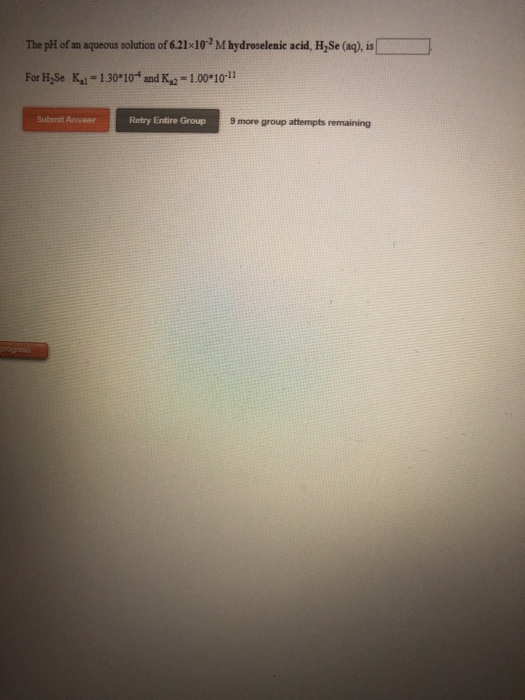The pH of an aqueous solution of 6.21x102 M hydroselenic acid, H, Se (aq), is For H Se K1=1.30*10and K 2 = 1.00*10-11 Submit Answer Retry Entire Group 9 more group attempts remaining Calculate the concentration of HS in an aqueous solution of 7.76x10-2M hydrosulfuric acid, H, (ag). [HS]- M Retry Entire Group 9 more group attempts remaining

• ### The pH of an aqueous solution of 0.2950 M carbonic acid, H2CO3 (aq), is Submit Answer...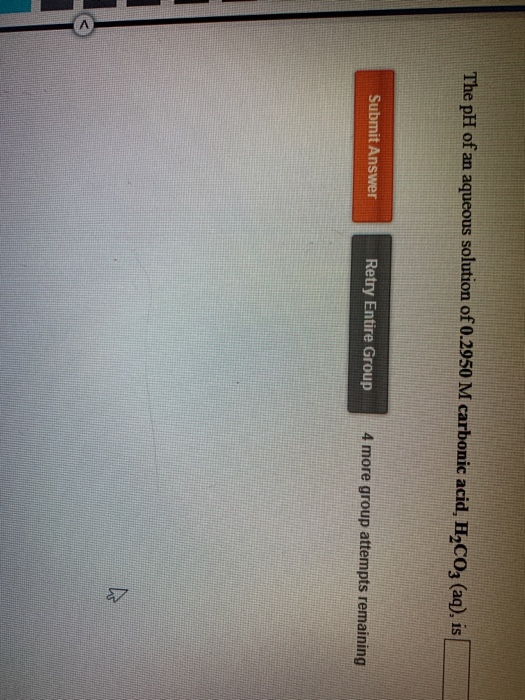The pH of an aqueous solution of 0.2950 M carbonic acid, H2CO3 (aq), is Submit Answer Retry Entire Group 4 more group attempts remaining

• ### Calculate the hydronium ion concentration in an aqueous solution of 0.165 M carbonic acid, H,CO, (aq)....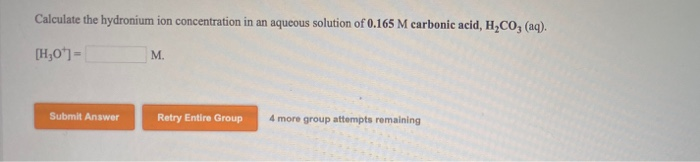Calculate the hydronium ion concentration in an aqueous solution of 0.165 M carbonic acid, H,CO, (aq). [H20"]= M. Submit Answer Retry Entire Group 4 more group attempts remaining

• ### Calculate the hydronium ion concentration in an aqueous solution of 4.86x10-2 Mascorbic acid, H,CH06 (aq). [H30*]=...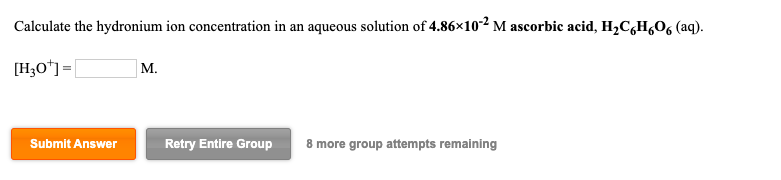Calculate the hydronium ion concentration in an aqueous solution of 4.86x10-2 Mascorbic acid, H,CH06 (aq). [H30*]= M. Submit Answer Retry Entire Group 8 more group attempts remaining Calculate the concentration of HCO3 in an aqueous solution of 0.2440 M carbonic acid, H2CO3(aq). [HCO3]= M. Submit Answer Retry Entire Group 8 more group attempts remaining

• ### The acid ionization constant for Sc(H20)63+(aq) is 5.0x10-5. Calculate the pH of a 0.0934 M solution...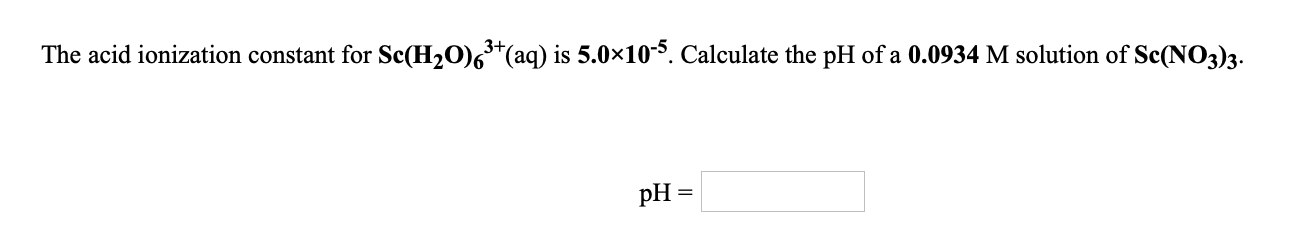The acid ionization constant for Sc(H20)63+(aq) is 5.0x10-5. Calculate the pH of a 0.0934 M solution of Sc(NO3)3. pH =

• ### The pH of an aqueous solution of 0.0640 M ascorbic acid, H,CH.O.(aq), is Submit Answer Retry...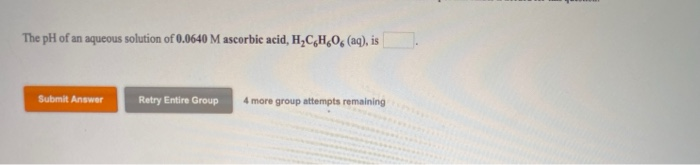The pH of an aqueous solution of 0.0640 M ascorbic acid, H,CH.O.(aq), is Submit Answer Retry Entire Group 4 more group attempts remaining

• ### 9 help! UWLV2 On X om Use the References to access important values if needed for...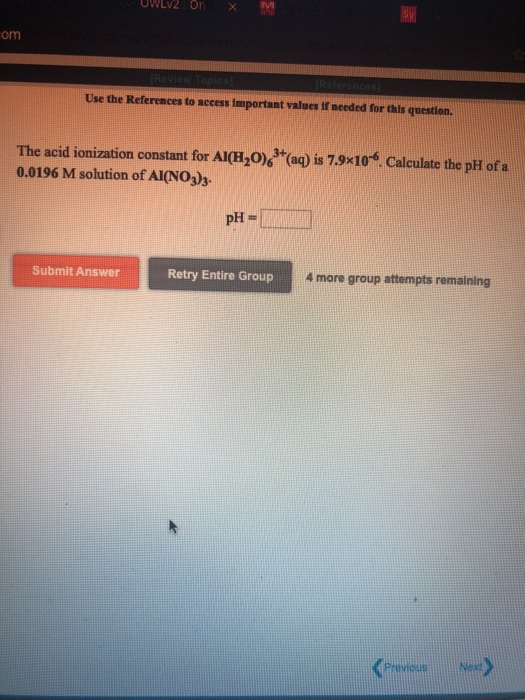9 help! UWLV2 On X om Use the References to access important values if needed for this question The acid ionization constant for Al(H20) + (aq) is 7.9x10. Calculate the pH of a 0.0196 M solution of AI(NO3)3. pH = Submit Answer Retry Entire Group 4 more group attempts remaining Previous Nex)

• ### Write the equations that represent the first and second ionization steps for sulfuric acid (H2SO4) in...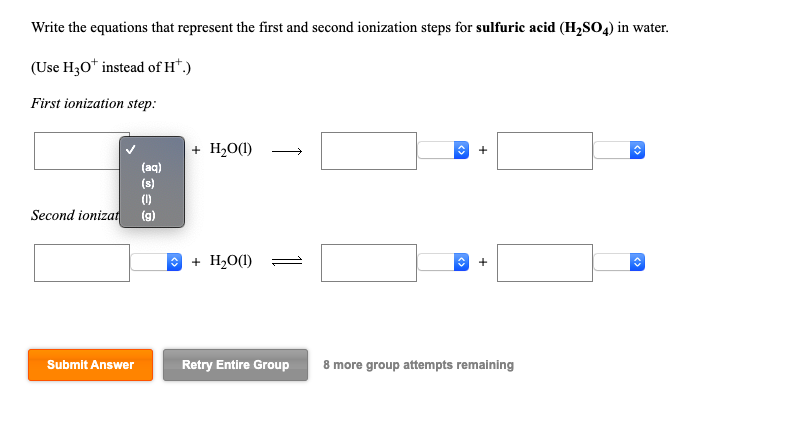Write the equations that represent the first and second ionization steps for sulfuric acid (H2SO4) in water. (Use H30+ instead of hf.) First ionization step: + H20() — + H2O(1) - + + (aq) Second ionizat (g) + H2O(1) + Submit Answer Retry Entire Group 8 more group attempts remaining Write the equations that represent the first and third ionization steps for arsenic acid (H2AsO4) in water. (Use Hz0+ instead of H.) first ionization step: + H20(1) = third ionization...

• ### Calculate the hydronium ion concentration in an aqueous solution of 4.95x10-2 Mascorbic acid, H,C6H 06 (aq)....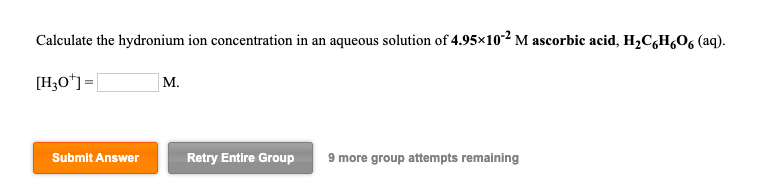Calculate the hydronium ion concentration in an aqueous solution of 4.95x10-2 Mascorbic acid, H,C6H 06 (aq). [H30*]= Submit Answer Retry Entire Group 9 more group attempts remaining Calculate the concentration of HCH.00 in an aqueous solution of 0.0713 M ascorbic acid, H,CH,06 (aq). [HC H/06)= M. Submit Answer Retry Entire Group 9 more group attempts remaining